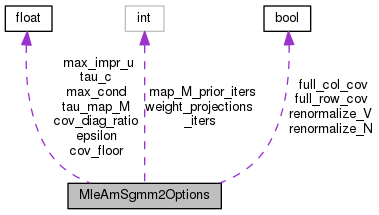MleAmSgmm2Options Struct Reference

Configuration variables needed in the SGMM estimation process. More...

`#include <estimate-am-sgmm2.h>`

Collaboration diagram for MleAmSgmm2Options:[legend]

## Public Member Functions

MleAmSgmm2Options ()

void Register (OptionsItf *opts)

## Public Attributes

BaseFloat tau_c
Smoothing constant for sub-state weights [count to add to each one]. More...

BaseFloat cov_floor
Floor covariance matrices Sigma_i to this times average cov. More...

BaseFloat cov_diag_ratio
ratio to dim below which we use diagonal. default 2, set to inf for diag. More...

BaseFloat max_cond
Max on condition of matrices in update beyond which we do not update. More...

bool renormalize_V

bool renormalize_N

int weight_projections_iters
Number of iters when re-estimating weight projections "w". More...

BaseFloat epsilon
very small value used to prevent SVD crashing. More...

BaseFloat max_impr_u
max improvement per frame allowed in update of u. More...

BaseFloat tau_map_M
For MAP update of the phonetic subspace M. More...

int map_M_prior_iters
num of iterations to update the prior of M More...

bool full_row_cov
Estimate row covariance instead of using I. More...

bool full_col_cov
Estimate col covariance instead of using I. More...

## Detailed Description

Configuration variables needed in the SGMM estimation process.

Definition at line 40 of file estimate-am-sgmm2.h.

## ◆ MleAmSgmm2Options()

 MleAmSgmm2Options ( )
inline

Definition at line 66 of file estimate-am-sgmm2.h.

66  {
67  cov_floor = 0.025;
68  tau_c = 2.0;
69  cov_diag_ratio = 2.0; // set this to very large to get diagonal-cov models.
70  max_cond = 1.0e+05;
71  epsilon = 1.0e-40;
72  renormalize_V = true;
73  renormalize_N = false; // default to false since will invalidate spk vectors
74  // on disk.
76  max_impr_u = 0.25;
77
79  tau_map_M = 0.0; // No MAP update by default (~500-1000 depending on prior)
80  full_row_cov = false;
81  full_col_cov = false;
82  }
BaseFloat cov_floor
Floor covariance matrices Sigma_i to this times average cov.
int map_M_prior_iters
num of iterations to update the prior of M
BaseFloat tau_c
Smoothing constant for sub-state weights [count to add to each one].
BaseFloat epsilon
very small value used to prevent SVD crashing.
bool full_row_cov
Estimate row covariance instead of using I.
BaseFloat cov_diag_ratio
ratio to dim below which we use diagonal. default 2, set to inf for diag.
int weight_projections_iters
Number of iters when re-estimating weight projections "w".
bool full_col_cov
Estimate col covariance instead of using I.
BaseFloat max_impr_u
max improvement per frame allowed in update of u.
BaseFloat max_cond
Max on condition of matrices in update beyond which we do not update.
BaseFloat tau_map_M
For MAP update of the phonetic subspace M.

## ◆ Register()

 void Register ( OptionsItf * opts )
inline

Definition at line 84 of file estimate-am-sgmm2.h.

References OptionsItf::Register().

Referenced by main().

84  {
85  std::string module = "MleAmSgmm2Options: ";
86  opts->Register("tau-c", &tau_c, module+
87  "Count for smoothing weight update.");
88  opts->Register("cov-floor", &cov_floor, module+
89  "Covariance floor (fraction of average covariance).");
90  opts->Register("cov-diag-ratio", &cov_diag_ratio, module+
91  "Minimum occ/dim ratio below which use diagonal covariances.");
92  opts->Register("max-cond", &max_cond, module+"Maximum condition number used to "
93  "regularize the solution of certain quadratic auxiliary functions.");
94  opts->Register("weight-projections-iters", &weight_projections_iters, module+
95  "Number for iterations for weight projection estimation.");
96  opts->Register("renormalize-v", &renormalize_V, module+"If true, renormalize "
97  "the phonetic-subspace vectors to have meaningful sizes.");
98  opts->Register("renormalize-n", &renormalize_N, module+"If true, renormalize "
99  "the speaker subspace to have meaningful sizes.");
100  opts->Register("max-impr-u", &max_impr_u, module+"Maximum objective function "
101  "improvement per frame allowed in update of u (to "
102  "maintain stability.");
103
104  opts->Register("tau-map-M", &tau_map_M, module+"Smoothing for MAP estimate "
105  "of M (0 means ML update).");
106  opts->Register("map-M-prior-iters", &map_M_prior_iters, module+
107  "Number of iterations to estimate prior covariances for M.");
108  opts->Register("full-row-cov", &full_row_cov, module+
109  "Estimate row covariance instead of using I.");
110  opts->Register("full-col-cov", &full_col_cov, module+
111  "Estimate column covariance instead of using I.");
112  }
BaseFloat cov_floor
Floor covariance matrices Sigma_i to this times average cov.
int map_M_prior_iters
num of iterations to update the prior of M
BaseFloat tau_c
Smoothing constant for sub-state weights [count to add to each one].
bool full_row_cov
Estimate row covariance instead of using I.
BaseFloat cov_diag_ratio
ratio to dim below which we use diagonal. default 2, set to inf for diag.
int weight_projections_iters
Number of iters when re-estimating weight projections "w".
bool full_col_cov
Estimate col covariance instead of using I.
BaseFloat max_impr_u
max improvement per frame allowed in update of u.
BaseFloat max_cond
Max on condition of matrices in update beyond which we do not update.
BaseFloat tau_map_M
For MAP update of the phonetic subspace M.

## ◆ cov_diag_ratio

 BaseFloat cov_diag_ratio

ratio to dim below which we use diagonal. default 2, set to inf for diag.

Definition at line 46 of file estimate-am-sgmm2.h.

## ◆ cov_floor

 BaseFloat cov_floor

Floor covariance matrices Sigma_i to this times average cov.

Definition at line 44 of file estimate-am-sgmm2.h.

## ◆ epsilon

 BaseFloat epsilon

very small value used to prevent SVD crashing.

Definition at line 58 of file estimate-am-sgmm2.h.

## ◆ full_col_cov

 bool full_col_cov

Estimate col covariance instead of using I.

Definition at line 64 of file estimate-am-sgmm2.h.

Referenced by TestSgmm2AccsIO().

## ◆ full_row_cov

 bool full_row_cov

Estimate row covariance instead of using I.

Definition at line 63 of file estimate-am-sgmm2.h.

Referenced by TestSgmm2AccsIO().

## ◆ map_M_prior_iters

 int map_M_prior_iters

num of iterations to update the prior of M

Definition at line 62 of file estimate-am-sgmm2.h.

## ◆ max_cond

 BaseFloat max_cond

Max on condition of matrices in update beyond which we do not update.

Should probably be related to numerical properties of machine or BaseFloat type.

Definition at line 50 of file estimate-am-sgmm2.h.

## ◆ max_impr_u

 BaseFloat max_impr_u

max improvement per frame allowed in update of u.

Definition at line 59 of file estimate-am-sgmm2.h.

## ◆ renormalize_N

 bool renormalize_N

Definition at line 53 of file estimate-am-sgmm2.h.

## ◆ renormalize_V

 bool renormalize_V

Definition at line 52 of file estimate-am-sgmm2.h.

## ◆ tau_c

 BaseFloat tau_c

Smoothing constant for sub-state weights [count to add to each one].

Definition at line 42 of file estimate-am-sgmm2.h.

## ◆ tau_map_M

 BaseFloat tau_map_M

For MAP update of the phonetic subspace M.

Definition at line 61 of file estimate-am-sgmm2.h.

Referenced by TestSgmm2AccsIO().

## ◆ weight_projections_iters

 int weight_projections_iters

Number of iters when re-estimating weight projections "w".

Definition at line 56 of file estimate-am-sgmm2.h.

The documentation for this struct was generated from the following file: